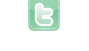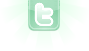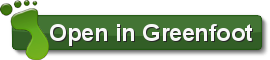Report as inappropriate.

# Cube3

The game I am making inorder to enter the monthly competetion.
I am still working on this, but this is the initial release.

Controls; W, A & D

This game is a work in progress, please be patient and report any bugs.

Thanks to danpost, Zamoht, davmac, MatheMagician & Gevater_Tod4711 for all the help they have given me.

Commence.

3702 views / 639 in the last 7 days

5 votes | 0 in the last 7 days

This scenario is a member of: Challenge WinnersYour browser does not support the canvas tag.
Thanks Dan, I think Kart is sleeping or is busy. Thanks for you scenario and all your help, once again!
A new version of this scenario was uploaded on Tue Jul 30 21:43:14 UTC 2013 Build: 1.13 Changelog: -Finished Cursor -Added Intro -Improved the Graphics *Give me feed-back on the background color and tell me if you find the easter egg. Here is a hint: 'power'.
;). P.S. Update coming soon!
I sometimes glitch into the blocks when I land and get stuck.
I've recently created a physics scenario that simulates gravity using a bouncing ball. I made collision detection for it that is 100% incapable of glitching into things (to my knowledge). You should take a look! http://www.greenfoot.org/scenarios/9172 Also, here is the code (to save you some trouble)! [code] import greenfoot.*; public class Ball extends Actor { int xmove=0; int ymove=0; boolean shadowImagery=false; boolean sdown=false; boolean gdown=false; public void act() { //Shadow Imagery Cheat if (Greenfoot.isKeyDown("s")&&sdown==false){ if (shadowImagery==false){shadowImagery=true;}else{shadowImagery=false;} sdown=true; }else if (! Greenfoot.isKeyDown("s")){sdown=false;} if (shadowImagery==true)getWorld().addObject(new Shadow(getImage()),getX(),getY()); //Zero Gravity Cheat if (Greenfoot.isKeyDown("g")&&gdown==false){ if (gravity==true){gravity=false;}else{gravity=true;} gdown=true; }else if (! Greenfoot.isKeyDown("g")){gdown=false;} //Method Calls grab(); physics(); } public void grab(){ MouseInfo mouse = Greenfoot.getMouseInfo(); if (mouse==null)return; if (mouse.getButton()>0){ if (mouse.getButton()==1){ if (getX()>mouse.getX()){xmove--;} if (getX()<mouse.getX()){xmove++;} if (getY()>mouse.getY()){ymove--;} if (getY()<mouse.getY()){ymove++;} } } } /**Copy everything between this blue comment and the next one to apply physics to an object (I will eventually apply elasticity properties so it is more realistic)*/ int gravityTimeRateMax = 3; //Amount of cycles until speed increases int gravityTimeRate = gravityTimeRateMax; int frictionAmountMax = 300; //How many cycles until the object slows //down due to friction int xFrictionAmount=0; int yFrictoinAmount=0; boolean gravity = true; //Just in case you want to switch gravity ;D Class[] objects = {Ball.class,Box.class}; //All objects that can be collided with public void gravity(boolean g){gravity=g;} public void physics(){ boolean ground = false; int collisionAmount=0; boolean xhold=false; boolean yhold=false; //Collision detection while (collisionAmount<objects.length){ //Down check for (int i=-getImage().getWidth()/2+4; i<getImage().getWidth()/2-4; i+=4){ Actor object = getOneObjectAtOffset(i, getImage().getHeight()/2+2+ymove,objects[collisionAmount]); if (object!=null&&ymove>=0){yhold=true; ground = true; ymove=-ymove/2; setLocation(getX(),object.getY()-object.getImage().getHeight()/2-getImage().getHeight()/2); break;} } //Up check for (int i=-getImage().getWidth()/2+4; i<getImage().getWidth()/2-4; i+=4){ Actor object = getOneObjectAtOffset(i, -getImage().getHeight()/2-3+ymove,objects[collisionAmount]); if (object!=null&&ymove<=0){yhold=true; ymove=-ymove/2; setLocation(getX(),object.getY()+object.getImage().getHeight()/2+getImage().getHeight()/2); break;} } //Left check for (int i=-getImage().getHeight()/2+4; i<getImage().getHeight()/2-4; i+=4){ Actor object = getOneObjectAtOffset(0-getImage().getWidth()/2-3+xmove, i,objects[collisionAmount]); if (object!=null&&xmove<=0){xhold=true; xmove=-xmove/2; setLocation(object.getX()+object.getImage().getWidth()/2+getImage().getWidth()/2,getY()); break;} } //Right check for (int i=-getImage().getHeight()/2+4; i<getImage().getHeight()/2-4; i+=4){ Actor object = getOneObjectAtOffset(getImage().getWidth()/2+2+xmove, i,objects[collisionAmount]); if (object!=null&&xmove>=0){xhold=true; xmove=-xmove/2; setLocation(object.getX()-object.getImage().getWidth()/2-getImage().getWidth()/2,getY()); break;} } collisionAmount++; } //Gravity if (ground==false&&gravity==true){ if (gravityTimeRate==0){ gravityTimeRate=gravityTimeRateMax; ymove++; }else{ gravityTimeRate--; } }else{gravityTimeRate=gravityTimeRateMax;} //Friction if (ground==true&&xFrictionAmount<frictionAmountMax&&xmove!=0){ xFrictionAmount++; } if (xFrictionAmount==frictionAmountMax||xmove==0){ xFrictionAmount=0; if (xmove>0)xmove--; if (xmove<0)xmove++; } //Move commands if (xhold==false)setLocation(getX()+xmove,getY()); if (yhold==false)setLocation(getX(),getY()+ymove); } /**End of code (don't forget to call the "physics" method)!*/ } [/code]
Sorry for taking up so much space...